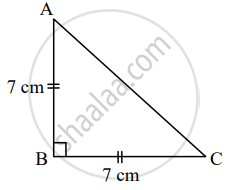# A congruent side of an isosceles right angled triangle is 7 cm, Find its perimeter - Geometry

Sum

A congruent side of an isosceles right angled triangle is 7 cm, Find its perimeter

#### SolutionGiven: In ∆ABC, ∠ABC = 90°, AB = BC = 7 cm

To find: Perimeter of ∆ABC

In ∆ABC, ∠ABC = 90°     ......[Given]

∴ AC2 = AB2 + BC2    ......[Pythagoras theorem]

∴ AC2 = (7)2 + (7)2

∴ AC2 = 49 + 49

∴ AC2 = 98

∴ AC = sqrt(49 xx 2)    ......[Taking square root of both sides]

Perimeter of ∆ABC = AB + BC + AC

= 7 + 7 + 7sqrt(2)

= 14 + 7sqrt(2) cm

= 7(2 + sqrt2)

∴ The perimeter of the given triangle is 7(2 + sqrt2) cm

Concept: Similarity in Right Angled Triangles
Is there an error in this question or solution?
Chapter 2: Pythagoras Theorem - Q.3 (B)

Share